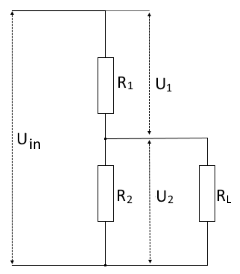Online calculator for calculating the values ​​on a loaded voltage divider

## Calculate the loaded voltage divider

This function calculates voltages, currents and resistances on a loaded voltage divider.

The values of the voltage U2 or the load resistance R3 can be specified at the output of the circuit. The input of the voltage U2 is preset.

 Input Total voltage mV V Resistor R1 mΩ Ω kΩ MΩ Resistor R2 mΩ Ω kΩ MΩ Voltage U2 Load resistor R3 mV V mΩ Ω kΩ MΩ Decimal places 0 1 2 3 4 6 8 10 Result Voltage U1 Voltage U2 Resistor R3 Resistor R2 || R3 Total resistance Current through R1 Current through R2 Current through R3## Formulas for the loaded voltage divider

The voltage divider is a series circuit made up of two resistors that divide an electrical voltage. When the voltage divider is loaded, a further resistor (load resistor) is connected in parallel to the second resistor. The ratio of the partial voltages corresponds to the ratio of the resistors R1 and the resistance of the parallel connection of (R2 and RL).

For the calculation, the total resistance of R2 and RL must therefore first be calculated using the following formula:

$$\displaystyle U_{2L}=\frac{R_2·R_3}{R_2+R_3}$$

Then the voltage U2 is calculated according to the formula:

$$\displaystyle U_2=U· \frac{R_{2L}} {R_1+R_{2L}}$$

The partial currents are calculated according to Ohm's law

$$\displaystyle I_1=\frac{U_1} {R_1}\;\;\;\;\;I_2=\frac{U_2} {R_2}\;\;\;\;\;I_3=\frac{U_2} {R_3}$$

 Ist diese Seite hilfreich?             Vielen Dank für Ihr Feedback! Wie können wir die Seite verbessern?